#### Polynomials

Suppose there arevariables,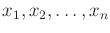. A monomial over a field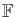is a product of the form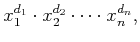(4.47)

in which all of the exponents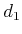,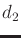,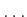,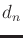are positive integers. The total degree of the monomial is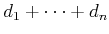.

A polynomial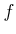in variables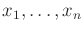with coefficients inis a finite linear combination of monomials that have coefficients in. A polynomial can be expressed as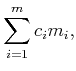(4.48)

in which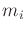is a monomial as shown in (4.47), and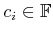is a coefficient. If, then each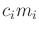is called a term. Note that the exponents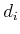may be different for every term of. The total degree ofis the maximum total degree among the monomials of the terms of. The set of all polynomials inwith coefficients inis denoted by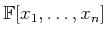.

Example 4..17 (Polynomials)   The definitions correspond exactly to our intuitive notion of a polynomial. For example, suppose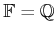. An example of a polynomial in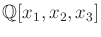is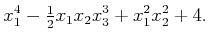(4.49)

Note thatis a valid monomial; hence, any element ofmay appear alone as a term, such as the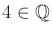in the polynomial above. The total degree of (4.49) is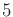due to the second term. An equivalent polynomial may be written using nicer variables. Using,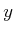, and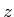as variables yields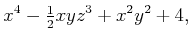(4.50)

which belongs to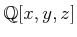.The setof polynomials is actually a group with respect to addition; however, it is not a field. Even though polynomials can be multiplied, some polynomials do not have a multiplicative inverse. Therefore, the setis often referred to as a commutative ring of polynomials. A commutative ring is a set with two operations for which every axiom for fields is satisfied except the last one, which would require a multiplicative inverse.

Steven M LaValle 2020-08-14